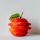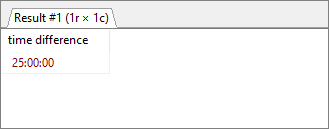Home
IT Knowledge
Inspiration
Languages
EN

# MySQL - difference between two datetimes

0 points
Created by:Khloe-Kaiser
398

In this article, we would like to show you how to calculate the difference between two datetimes in MySQL.

Quick solution:

``````-- SELECT TIMEDIFF('to_time','from_time');
SELECT TIMEDIFF('YYYY-MM-DD HH:MM:SS','YYYY-MM-DD HH:MM:SS');``````

Where:

• `YYYY` - four-digit year format, e.g. `2021`,
• `MM` - month number (counted from `01` to `12`),
• `DD` - day number (from `01` to `31` - depending on month),
• `HH` - hour number (from `00` to `24`),
• `MM` - minute number (from `00` to `59`),
• `SS` - second number (from `00` to `59`).

## Practical example

In this example, we will calculate the difference between two datetimes using `TIMEDIFF()` function.

Query:

``SELECT TIMEDIFF('2021-01-09 09:00:00','2021-01-08 08:00:00') AS 'time difference';``

Result:MySQL - result of computing the difference between two datetimes (25h)

Note:

If you only want to calculate the difference between days (dates), go to this article.